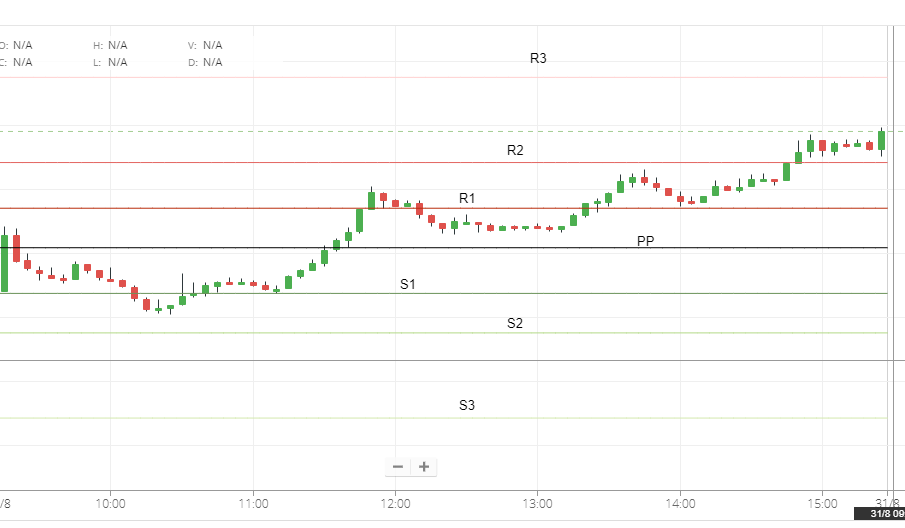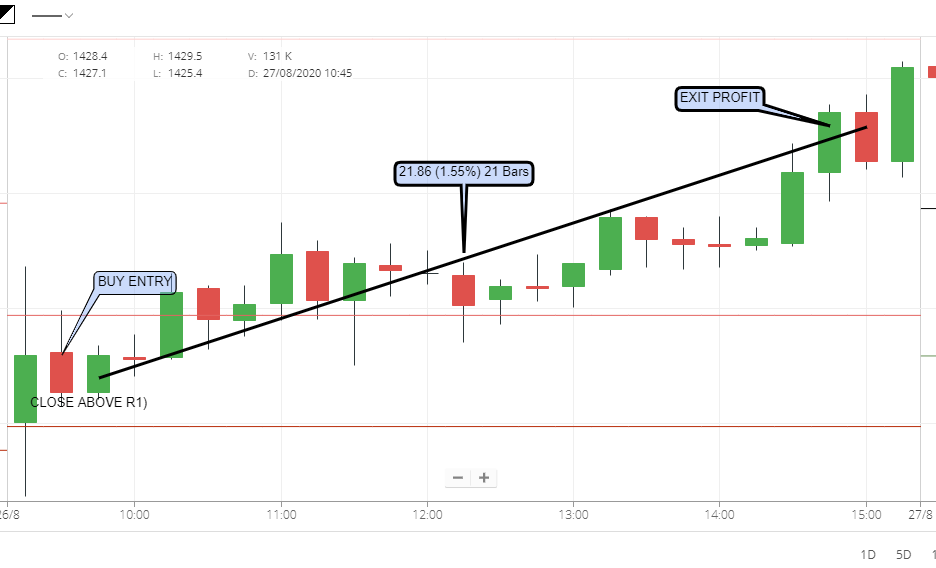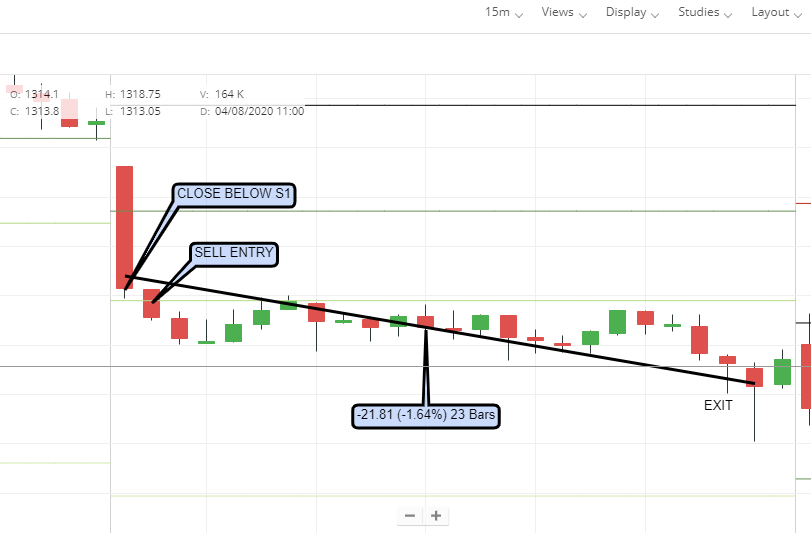Here in this topic, we discuss pivot point intraday trading strategy & pivot technical support and resistance. The pivot point indicator is a very important technical indicator, to calculate technical levels. Now here we discussed various uses of pivot point indicators for intraday trading.

Before talking about the Pivot point intraday trading strategy using the pivot point indicator first we show you the mathematical formula of technical pivot point indicator.

Pivot point intraday trading strategy & Pivot point is a mathematical tool to calculate support and resistance and it is calculated with the help of high low previous close and open price.

# The pivot point calculation is shown below:

Pivot Point: (P) = (High + Low + Close)/3
Support 1 (S1) = (P x 2) – High
Support 2 (S2) = P  –  (High  –  Low)
(R1) = (P x 2) – Low
(R2) = P + (High  –  Low)

### Pivot strategy

#### Pivot strategy:  In Pivot point intraday trading strategy: trading we need a basic candle chart with pivot point indicator setup, shown below :the pivot point is basically a mathematical technical indicator used to predict the current day support and resistance with the help of previous day open, high, low, and close price.
pivot point works with different time periods and gives a result as per the time frame in the technical chart.

#### Type of pivot point indicator

basically, two types of pivot point indicators are used in the technical chart.

#### 1. standard pivot point indicator

this is the standard pivot point indicator easy to calculate mathematically with the help of previous day high low close and open prices.

here the formula to calculate pivot point is.

 The pivot point(PP) (High+Low+Close)/3 Resistance(R1) 2*PP-LOW Resistance(R2) PP+(HIGH-LOW) Resistance(R3) High+2*(PP-Low) Support(S1) 2*(PP-HIGH) Support(S2) PP-(HIGH-LOW) Support(S3) low-2*(High-PP

#### 2.Fibonacci pivot points

the Fibonacci pivot point is the most popular technical pivot indicator, the Fibonacci mostly used for retracement trading in the Fibonacci pivot point indicator basically used to project or predict to the next support and resistance levels. in the Fibonacci pivot indicator, the trading accuracy is more than a simple pivot indicator that's why the Fibonacci pivot indicator is most widely used technical Fibonacci pivot indicator.

the Fibonacci 38.20% and 61.80 % levels are the most important retracement levels.

 pivot point mathematical formula Pivot Point (P) (High + Low + Close)/3 upport 1 (S1) P – {.382 * (High – Low)} Support 2 (S2) P – {.618 * (High – Low)} Support 3 (S3) P – {1 * (High – Low)} Resistance 1 (R1) P + {.382 * (High – Low)} Resistance 2 (R2) P + {.618 * (High – Low)} Resistance 3 (R3) P + {1 * (High – Low)}

#### The Woodie's pivot point

in woddie pivot point indicator is totally focus on the previous day closing price. here below the woodie pivot point mathematical formula shows.

 pivot point mathematical formula Pivot Point (P) (High + Low) + (2 x Closing Price) / 4 Resistance 1 (R1) (2 X PP) – Low Resistance 2 (R2) PP + (High – Low) support 1 (S1) PP + (High – Low) Support 2 (S2) PP – (High + Low)

#### Camarilla pivot points

the nick Scott camarilla is an inventor of the camarilla pivot point indicator.in a camarilla pivot point indicator, there are 4 supports and 4 resistance levels.
it is very similar to a woddies pivot point indicator.

below the mathematical formula of the camarilla pivot point indicator.

 pivot point mathematical formula Pivot Point (P) (High + Low + Closing) / 3 support 1 (S1) Closing – ((High -Low) x 1.0833) Support 2 (S2) Closing – ((High -Low) x 1.1666) Support 3 (S3) Closing – ((High -Low) x 1.2500) Support 4 (S4) Closing – ((High-Low) x 1.5000 Resistance 1 (R1)0 Closing + ((High -Low x 1.0833) Resistance 2 (R2) Closing + ((High -Low) x 1.1666) Resistance 3 (R3) Closing + ((High -Low) x 1.2500) Resistance 4 (R4) Closing + ((High -Low) x 1.5000)

### Demark Pivot Points

Tom demark has invented this pivot point indicator for trading purposes. in this pivot point basically use of open and high price to find the proper supports and resistance.

there is three conditions to find out pivot and support resistance, levels.

If Close > Open, then X = (2 x High) + Low + Close

If Close < Open, then X = High + (2 x Low) + Close

If Close = Open, then X = High + Low + (2 x Close)

 pivot point mathematical formula Pivot Point (P) X/4 Resistance 1 (R1) X/2 – Low support 1 (S1) X/2 – High

### pivot point working

in a candle chart or any type of historical price chart the pivot point indicator draws the supported price and resistive price line.

when the price action happens and the price reached the support or resistance levels the intraday trader makes the position accordingly and trade with proper pivot discipline.
so the pivot point indicator basically provides us a trading discipline and also provides entry and exit price in the form of pivot support and resistance levels.

\

so here we use the first candle chart and pivot point indicator in the intraday trading chart with 15-minute time frame of candle chart, and buy only those stock after the first 15-minute candle close above the R1( resistance1), and sell only when the first 15-minute candle closes below the S1(supports 1). We need to enter our intraday trade after the first 15-minute candle closes or the opening price of another candle. Similarly sell the stock when the first candle closes or the opening of a new candle.

# Pivot point intraday trading strategy at buy condition when a 15-minute first candle closes above the resistance 1(R1).PIVOT POINT BUY CONDITION

Pivot point intraday trading strategy in sell condition when a 15-minute first candle closes Below the support 1(S1).PIVOT POINT SELL CONDITION

2.Full day trade with this strategy and exit at the closing of the market.
3. Some time big profits happen.

1. Some time market falls from high and closes near low.
2.its works only 70% of cases.

1.1.2.